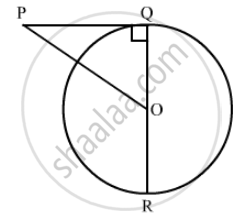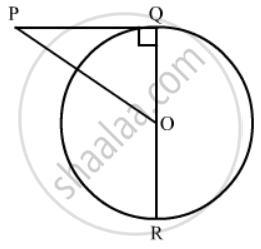Advertisement Remove all ads

# In the Given Figure, Oq : Pq = 3.4 and Perimeter of δ Poq = 60 Cm. Determine Pq, Qr and Op. - Mathematics

Short Note

In the given figure, OQ : PQ = 3.4 and perimeter of Δ POQ = 60 cm. Determine PQ, QR and OP.Advertisement Remove all ads

#### Solution

In the figure,∠ PQD. Therefore we can use Pythagoras theorem to find the side PO.

PO^2=PQ^2+OQ^2 …… (1)

In the problem it is given that,

(OQ)/(PQ)=3/4

OQ= 3/4PQ.....(2)

Substituting this in equation (1), we have,

PQ^2=(9PQ^2)/16+PQ^2

PQ^2=(25PQ^2)/16

PQ^2=sqrt((25PQ^2)/16)

PQ=5/4PQ........(3)

It is given that the perimeter of  Δ POQis 60 cm. Therefore,

PQ + OQ + PO = 60

Substituting (2) and (3) in the above equation, we have,

PQ+3/4PQ+5/4PQ=60

12/4PQ=60

3PQ=60

PQ=20

Substituting for PQ in equation (2), we have,

PD=5/4xx15

OQ=3/4xx20

OQ=15

OQ is the radius of the circle and QR is the diameter. Therefore,

QR = 2OQ

QR = 30

Substituting for PQ in equation (3), we have,

PD=5/4xx20

PO=25

Thus we have found that PQ = 20 cm, QR = 30 cm and PO = 25 cm.

Is there an error in this question or solution?
Advertisement Remove all ads

#### APPEARS IN

RD Sharma Class 10 Maths
Chapter 8 Circles
Exercise 8.2 | Q 44 | Page 40
Advertisement Remove all ads
Advertisement Remove all ads
Share
Notifications

View all notifications

Forgot password?